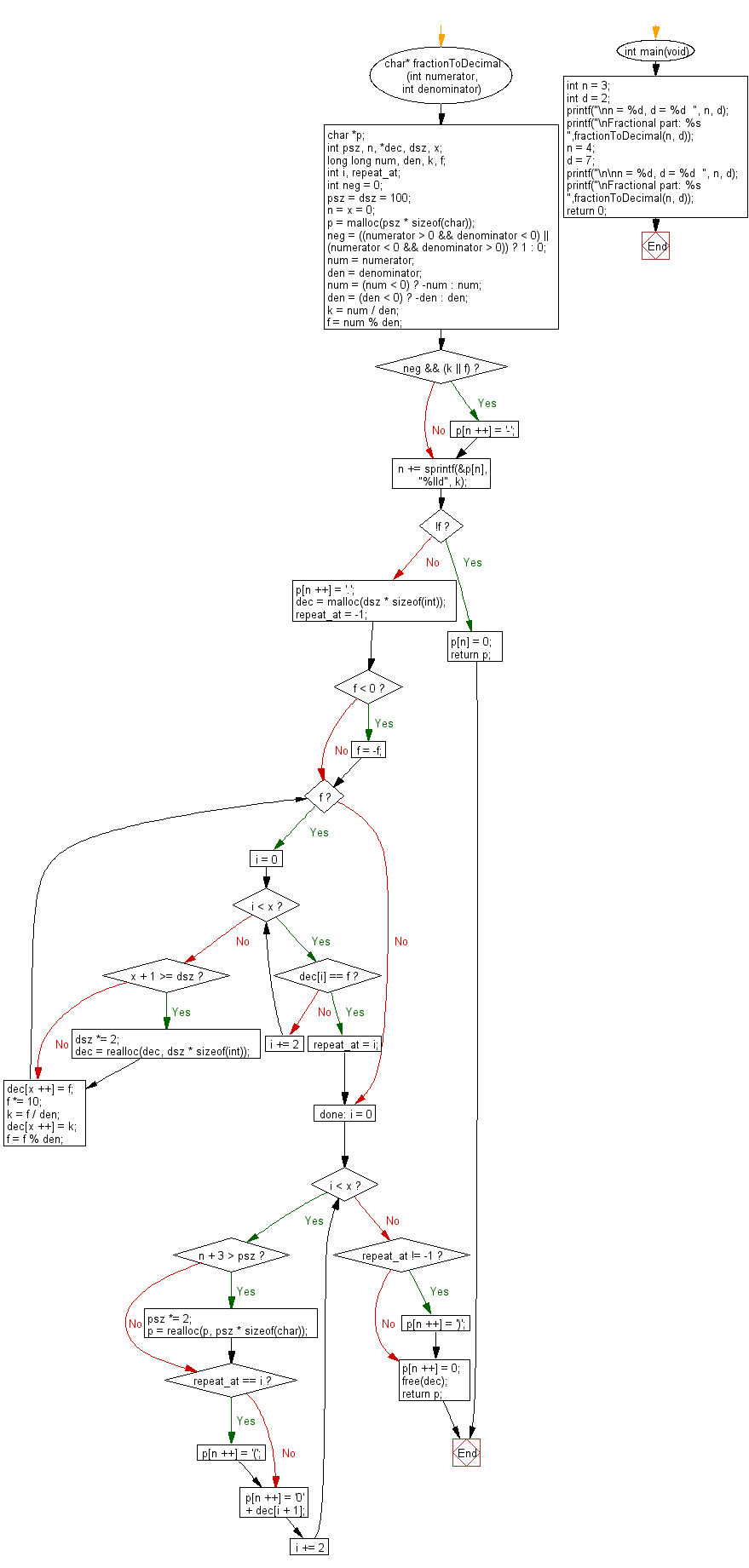﻿ C Program: Fraction part of two integers in string

# C Exercises: Get the fraction part from two given integers representing the numerator and denominator in string format

## C Programming Mathematics: Exercise-7 with Solution

Write a C program to get the fraction part from two given integers representing the numerator and denominator in string format.

Example:
Input:
n = 3
d = 2

n = 4
d = 7
Output:
Fractional part: 1.5
Fractional part: 0.(571428)

Sample Solution:

C Code:

``````//Source: https://bit.ly/2KNsta8
#include <stdio.h>
#include <stdlib.h>
#include <string.h>
#include <stdbool.h>

char* fractionToDecimal(int numerator, int denominator) {
char *p;
int psz, n, *dec, dsz, x;
long long num, den, k, f;
int i, repeat_at;
int neg = 0;

psz = dsz = 100; n = x = 0;
p = malloc(psz * sizeof(char));
//assert(p);

neg = ((numerator > 0 && denominator < 0) ||
(numerator < 0 && denominator > 0)) ? 1 : 0;
num = numerator;
den = denominator;
num = (num < 0) ? -num : num;
den = (den < 0) ? -den : den;

k = num / den;
f = num % den;

if (neg && (k || f)) p[n ++] = '-';

n += sprintf(&p[n], "%lld", k);
if (!f) {
p[n] = 0;
return p;
}

p[n ++] = '.';

dec = malloc(dsz * sizeof(int));
//assert(dec);

repeat_at = -1;
if (f < 0) f = -f;
while (f) {
for (i = 0; i < x; i += 2) {
if (dec[i] == f) {
repeat_at = i;
goto done;
}
}
if (x + 1 >= dsz) {
dsz *= 2;
dec = realloc(dec, dsz * sizeof(int));
//assert(dec);
}
dec[x ++] = f;
f *= 10;
k = f / den;
dec[x ++] = k;
f = f % den;
}

done:
for (i = 0; i < x; i += 2) {
if (n + 3 > psz) {
psz *= 2;
p = realloc(p, psz * sizeof(char));
//assert(p);
}
if (repeat_at == i) {
p[n ++] = '(';
}
p[n ++] = '0' + dec[i + 1];
}
if (repeat_at != -1) p[n ++] = ')';
p[n ++] = 0;

free(dec);

return p;
}

int main(void)
{
int n = 3;
int d = 2;
printf("\nn = %d, d = %d  ", n, d);
printf("\nFractional part: %s ",fractionToDecimal(n, d));
n = 4;
d = 7;
printf("\n\nn = %d, d = %d  ", n, d);
printf("\nFractional part: %s ",fractionToDecimal(n, d));
return 0;
}
```
```

Sample Output:

```n = 3, d = 2
Fractional part: 1.5

n = 4, d = 7
Fractional part: 0.(571428)
```

Flowchart:C Programming Code Editor:

Improve this sample solution and post your code through Disqus.

What is the difficulty level of this exercise?

Test your Programming skills with w3resource's quiz.

﻿